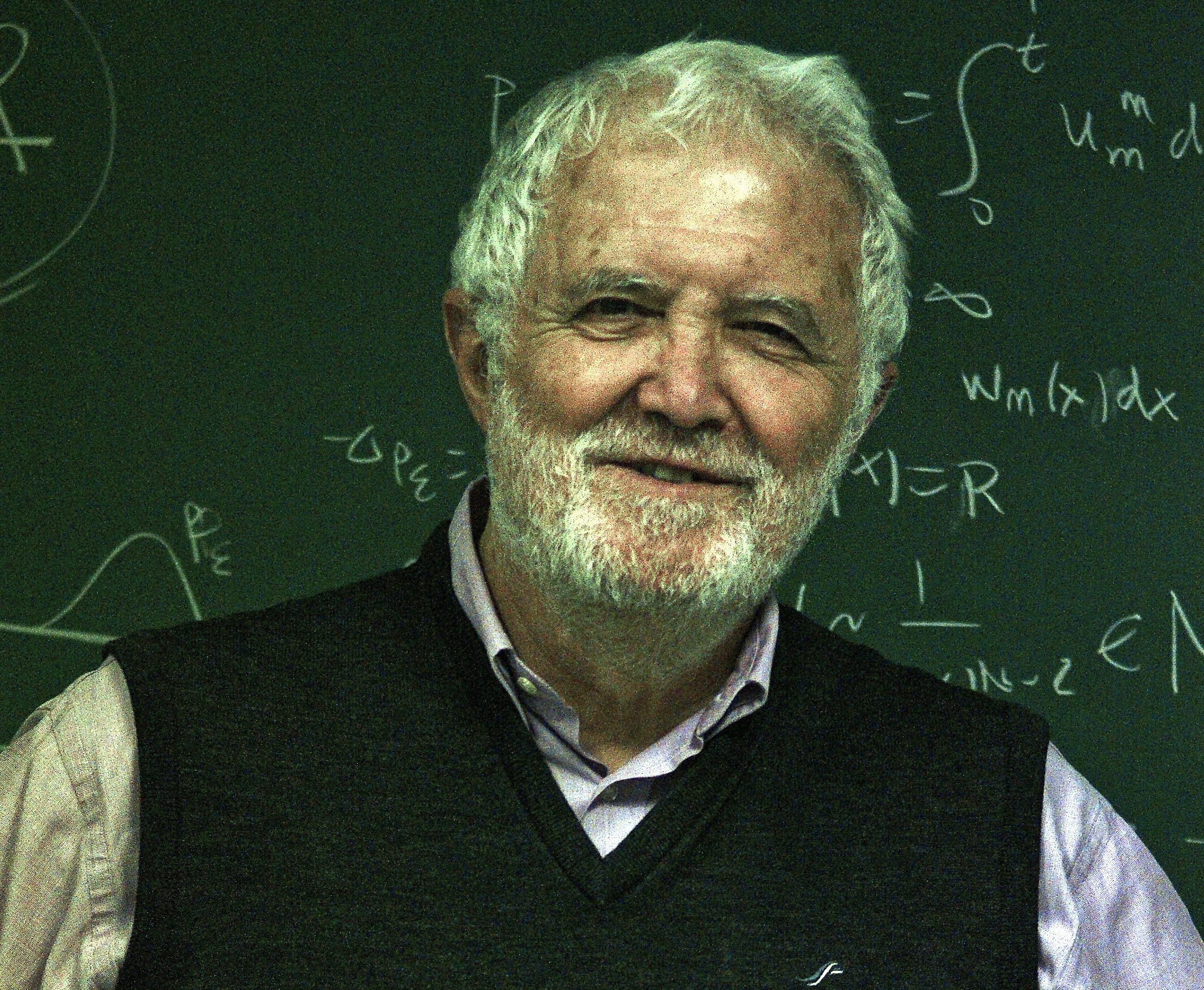# Vortex formation for a non-local interaction model with Newtonian repulsion and superlinear mobility

Published on by Jose Carrillo
We consider density solutions for gradient flow equations of the Newtonian repulsive interaction energy in the whole space $\Rd$ with the nonlinear convex mobility $\gamma(u)=u^\alpha$, and $\alpha>1$. We show that solutions corresponding to compactly supported initial data remain compactly supported for all times leading to moving free boundaries as in the linear mobility case $\gamma(u)=u$. Our present results are in sharp contrast with the case of concave mobilities of the form $\gamma(u)=u^\alpha$, with $0<\alpha<1$. We develop a well-posedness theory of viscosity solutions of the mass equation for radially symmetric initial data that leads to a very detail analysis allowing us to show a waiting time phenomena. This is a typical behavior for nonlinear degenerate diffusion equations like the porous medium equation. We also construct explicit self-similar solutions exhibiting similar vortex-like behaviour characterizing the long time asymptotics of general radial solutions under certain assumptions. Convergent numerical schemes based on the viscosity solution theory are proposed analysing their rate of convergence. The numerical simulations in this project illustrate the proven results in the arxiv preprint .

cite all items

## Funding

European Research Council Advanced Grant Nonlocal-CPD (Nonlocal PDEs for Complex Particle Dynamics: Phase Transitions, Patterns and Synchronization) under the European Union’s Horizon 2020 research and innovation programme (grant agreement No. 883363).

EPSRC grant number EP/P031587/1

email

## Members (3)Jose CarrilloDavid Gomez-CastroJuan Luis Vazquez